### Shapes Worksheets

A great way to learn circles, ovals, rectangles, squares, and triangles.

## What Are the Basic Geometric Shapes?

Everything in the world around us has a shape which is why geometry is so important and one of the classical disciplines of mathematics. In geometric terms, the form of an object and its outline or outer boundary can be defined as shape.

Once you have a basic understanding of the geometric shapes, you'll notice them in the world around you, such as coins, windows, toys, buildings, picture frames, etc. The list is endless. There are many applications of geometry in the real world, so learning the geometric shapes is essential.

If you are wondering what the basic geometric shapes are, we've got you covered. This blog will give you an understanding of what geometry is, the reasons for its importance, and the basic geometric shapes that you must know about! Read on to find out.

What is Geometry?

Geometry is essential for creating a solid foundation for advanced mathematical understanding as it often overlaps with algebra.

In simple terms, geometry is a branch of math that deals with the shapes of objects, the special relationship between those objects, and the properties of the space surrounding the objects.

Understanding shapes and the special relationship between them is essential for students, and it teaches them not only to draw shapes but also to measure and compare them. Geometry has many practical applications, from building houses to bridges, making it essential.

Understanding geometry will also help students think in terms of shapes and sizes, and they will be able to visualize the things around them in terms of the shapes they have learned.

Why is It So Important in Our Lives?

1. Developing Spatial Awareness

Geometry helps understand spatial relationships and creates a clear perception in students' minds regarding space in terms of the sizes and shapes of the objects around us. Spatial awareness defines the ability of a person to recognize how everything around them interacts with the space in which it exists and be able to fit things in the environment.

Spatial awareness is essential for understanding the shapes around us. In simpler terms, geometry helps in representing all the objects around us in the world and their inter-relationships

According to a study conducted by the University of Michigan, special awareness helps in improving students' understanding of critical mathematical concepts allowing them to perform better.

2. Understanding Measurements

Understanding geometric shapes allow you to deal with measurements and relationships between surfaces, lines, angles, and solids.

Understanding how things are measured is an essential skill with many practical applications, such as measuring a carpet or the window for curtains.

3. Engages Both Sides of Your Brain

The left side of your brain is more technical and logical, while the ride side is more creative. Geometry engages both sides of the brain to allow full use of brain capacity. It allows you to be both a left-brain thinker and a right-brain thinker.

4. Think Out of the Box

An understanding of geometric shapes allows you to think out of the box by applying these concepts to come up with solutions to problems in day-to-day situations. It allows you to think logically, making things easy to understand.

Basic Geometric Shapes

Students must know basic geometric shapes to form a solid foundational base for other mathematical concepts. Practice drawing these shapes and try to identify them in the world around you to understand the practical nature of geometry as it exists in all objects around you.

Here are the basic 2D or two-dimensional basic geometric shapes. These shapes are flat and have a length and width that allows you to measure them.

1. Circle

A circle is a round-shaped geometric shape that has one curved line, and all the lines on that line have the same distance from the center of the circle.

A circle does not have any sides, cones, or edges. It is a closed 2D shape.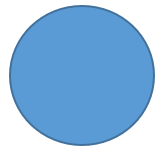2. Triangle

A triangle is a two-dimensional shape containing three straight sides. To form a triangle, three lines have to meet. A triangle can have three, two, or no sides of equal lengths.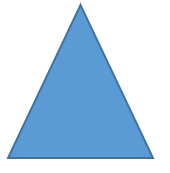3. Square

A square has four equal sides and four right angles (angles that measure 90 degrees). All four sides of a square are equal in length. The opposite sides of a square are both parallel.4. Rectangle

A rectangle is a 2D shape with four parallel and equal opposite sides. All the angles in the interior of the rectangle are 90° or right angles. All squares are rectangles since they have four sides. However, not all rectangles can be squares since the sides need to be equal, which is not true for all rectangles.5. Parallelogram

A parallelogram has two sets of parallel sides and two sets of sides that are equal, similar to a rectangle. The difference is that the interior angles are not right angles. Hence, we can conclude that all rectangles and squares are parallelograms but not vice versa.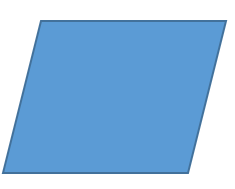6. Rhombus

A rhombus is a geometric shape with four equal sides. Since it has two parallel sets of sides, it is also a parallelogram. It is shaped like a diamond.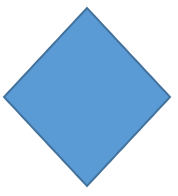7. Pentagon

A pentagon is a flat 2D shape with five sides and five vertices. All the internal angles of a pentagon add up to 540 degrees. It is enclosed, and all interior angles measure 108 degrees while exterior angles measure 72 degrees.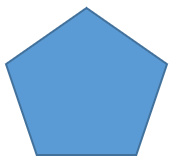8. Hexagon

A hexagon is a side-sided 2D flat geometric shape. In a hexagon, all the sides and interior angles are equal. Each interior angle in a hexagon measures 120 degrees.Concluding Thoughts

Geometry has several benefits for students and helps them understand and interact with the shapes around them. Understanding the geometric shapes can help students identify structures and symmetries quickly. They develop improved reasoning and logical thinking ability by applying the concepts they have learned in the world around them.

We are surrounded by space and the shapes of things. The planet we live on and the objects we see around us all have shapes that you can link to the study of geometry.

Hence, mastering geometric shapes will help you better understand the world of shapes! Keep practicing.